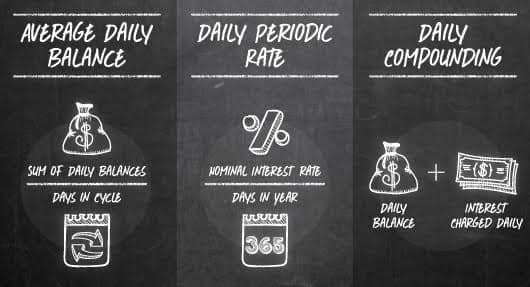# How to Find “Nominal” Interest Rate | A User-Friendly GuideGUIDEUpdated: May 7, 2018
Our popular “How-To” series is for those who seek to improve their subprime credit rating. Our articles follow strict editorial guidelines.

The nominal interest rate, also called the annualized percentage rate (APR), is the annual interest you pay for debt or receive for savings before accounting for inflation.

It’s important to know the nominal interest rate of credit cards and loans so you can identify the lowest-cost ones in a standardized way. It’s also important to distinguish nominal interest rate from real interest rate, which accounts for the erosion of buying power caused by inflation.

Lucky for you, we’ve written up this user-friendly guide. Let’s start with the equation.

## Nominal Interest Rate Equation:

 NIR = RIR + IR

NIR = nominal interest rate

RIR = real interest rate

IR = inflation rate

The same equation can be rewritten to find the real interest rate you’re paying or receiving:

 RIR = NIR – IR

## Example:

Imagine a credit card has a 15 percent APR, which is its NIR. You can find the current inflation rate by looking up the 12-month change in the Consumer Price Index (CPI). This index represents the monthly change in cost of a standard basket of goods and services.

If the CPI is zero, then the NIR is also the RIR. A 1 percent inflation rate translates to a 14 percent RIR:

RIR = NIR – IR = 15% – 1% = 14%

As you can see, the higher the inflation rate, the less costly the loan in real terms.

## How NIR is Used:

You have to understand these three concepts to see how NIR is used:

### 1. Average daily balance

This is the average amount you owe in the monthly billing cycle. It’s the sum of the daily balances divided by the days in the cycle.

### 2. Daily periodic rate

This is the interest rate applied each day to the daily balance. It is equal to the NIR (APR) divided by the number of days in the year (banks use 360 or 365). For example, an NIR of 15 percent and a 365-day year is equal to a 0.041 percent daily periodic rate.

### 3. Daily compounding

The interest charged each day is added to the daily balance to get the next day’s daily balance. This means you are paying interest on interest until your pay off your balance. NIR does not include the effect of compounding.

Because of compounding, your actual interest rate (effective APR) will exceed the NIR.You have to understand these three concepts to see how NIR is used.

## Example:

Your credit card charges a 15 percent APR/NIR and has a 30-day billing period. Suppose you owe \$1,000 on Day 1, pay \$300 on Day 11 and \$500 on Day 21, leaving your balance at \$200. Your average daily balance is:

(10 days x \$1,000 + 10 days x 700 + 10 days x \$200)/30 days = \$633

To compute your interest charge for the month, multiply your average daily balance by the daily periodic rate and multiply by the 30 days in the period:

\$633 x 0.041% x 30 = \$7.79

You can also use an online calculator to figure how much you will be paying if you make only minimum payments each month. The results can be astounding.

See, finding the nominal interest rate isn’t so tough! Hopefully by now you have a better understanding of nominal interest rates and are able to calculate yours with ease.